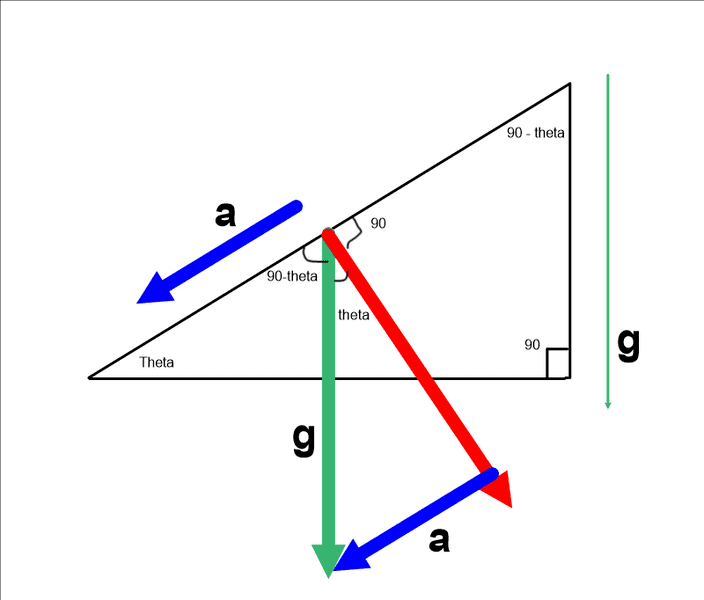# Component resolution of g on an inclined plane

themselv

## Homework Statement

Hi, I just had a quick conceptual question.
I understand that if I have an object moving down a ramp, it is being accelerated only by gravity. My question is this:

We should be able to experimentally calculate g if we measure the acceleration down the ramp. Then we can use trigonometry to determine a value for g. However, this only works if you take the
acceleration down the ramp divided by the sin (theta) . I understand where this comes from (redrawing the angle theta in the middle where the object starts from), but why can we not calculate the component of gravity by saying:

sin (theta) = g / a

This makes more sense to me looking at the initial angle, because g appears to be the opposite side and the acceleration down the ramp appears to be the hypotenuse.

Crake

## Homework Statement

Hi, I just had a quick conceptual question.
I understand that if I have an object moving down a ramp, it is being accelerated only by gravity. My question is this:

We should be able to experimentally calculate g if we measure the acceleration down the ramp. Then we can use trigonometry to determine a value for g. However, this only works if you take the
acceleration down the ramp divided by the sin (theta) . I understand where this comes from (redrawing the angle theta in the middle where the object starts from), but why can we not calculate the component of gravity by saying:

sin (theta) = g / a

This makes more sense to me looking at the initial angle, because g appears to be the opposite side and the acceleration down the ramp appears to be the hypotenuse.

## The Attempt at a Solution

I think it will be easier if you draw a diagram

themselvI realize that an expression for g should be g = a/sin(theta) by looking at the angle that's formed perpendicular to the plane.

However, if I just look at angle theta in the bottom left corner, why am I not justified in saying g = sin(theta)*a, since to me it appears that the sine of angle theta is just opposite over hypotenuse, or g/a.

Alexander83
"However, if I just look at angle theta in the bottom left corner, why am I not justified in saying g = sin(theta)*a, since to me it appears that the sine of angle theta is just opposite over hypotenuse, or g/a."

Because you've not got the correct triangle set up. The hypoteneuse of a triangle is its longest side. The component of the gravitational acceleration (g) in the down incline direction is what gives you a. Since a component (a) can never exceed the full vector (g) in magnitude, it follows that a cannot be the hypoteneuse.

Chris.